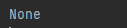# 如何在 Python 中计算平方根

Python 为我们提供了平方根的内置函数，它是数学模块的一部分。 要找到一个数字的平方根，我们首先导入数学模块，然后我们可以使用平方根函数。

## 平方根函数：

### 句法：

`math. sqrt(n)`

### 示例 1：

```import math

print("The square root of 36 is:",math.sqrt(36))```

36 的平方根是：6.0

### 示例 2：

```import math

n=eval(input("Enter a number to calculate square root"))

print("The square root of number is:",math.sqrt(n))```

Enter 计算平方根的数字 45### 示例 3：

```from math import sqrt

def pythagoras(x, y):

if x<= 0 or y <= 0:

return

return sqrt(x * x + y * y)

print(pythagoras(7, 4))```

8.06225774829855### 示例 4：

```from math import sqrt

def pythagoras(x, y):

if x<= 0 or y <= 0:

return

return sqrt(x * x + y * y)

print(pythagoras(-7, 4))```